# John-Deere Reasoning Question

1. In a certain code RAJAN is coded as '91115' . How is IQBAL written in that code?
1. 98213
2. 27582
3. 12968
4. 18213
5. None of these
The question is related to the position of the letter in English alphabet. Since R comes at 18th position so its code is 1+8 = 9. A comes at 1st position and since it is a single digit number so it is coded as 1.similarly J = 10 is coded as 1+0=1 and A as 1and N=14 as 1+4 =5. So code for RAJAN is 91115.
Similarly code for IQBAL is
I = 9
Q = 17 = 1 +7 = 8
B = 2
A = 1
L = 12 = 1+2 = 3
Therefore, 98213 is required option.
Hence option A is the answer
DIRECTIONS for the question 2 - 3:  Given an input line; the machine arranges the words and numbers in steps in a systematic manner as illustrated afterwards : Study the pattern and answer the question that follows.
Given an input line, a coding machine rearranges the input following certain steps as explained below:
Input: 47 desert go 56 there often 32 12
Step I: there 47 desert go 56 often 32 12
Step II: there 12 47 desert go 56 often 32
Step III: there 12 often 47 desert go 56 32
Step IV: there 12 often 32 47 desert go 56
Step V: there 12 often 32 go 47 desert 56
The arrangement in Step V is the final arrangement and Step V is the last step.
In each of the following questions the rearrangement is done following the same rules as explained in the above illustratio
2. If the fourth step of an input is 'wonderful 22 seashore 36 48 fine 62 morning', what was the first step?
1. fine 48 wonderful 22 seashore 36 62 morning
2. fine 48 wonderful 22 36 seashore 62 morning
3. fine 48 seashore wonderful 22 36 morning 62
4. fine 48 seashore wonderful 36 22 morning 62
5. Cannot be determined.
The logic followed in the given sequence is " numbers are arranged in increasing order while words are arranged in decreasing order of the first alphabet "
Since it is a case of arrangement ,so we cannot go from any of the step to any of backward step. Hence we cannot find the first step from fourth step. So option E is the answer.
1. What will be the third step for the following input?
Input: paper common 36 51 pencil 28 test 66
?
1. test 28 paper pencil common 36 51 66
2. test 28 pencil 66 paper common 36 51
3. test 66 pencil paper 28 common 51 36
4. test 28 pencil paper common 36 51 66
5. None of these
The logic followed in the given sequence is " numbers are arranged in increasing order while words are arranged in decreasing order of the first alphabet "
Input: paper common 36 51 pencil 28 test 66.
Step I: test paper common 36 51 pencil 28 66
Step II: test 28 paper common 36 51 pencil 66
Step III: test 28 pencil paper common 36 51 66.
Hence option D is the answer.
DIRECTIONS for the question 4:  The question below contains four statements, followed by four conclusions numbered I, II, III & IV. You have to take the given statements to be true even if they seem to be at variance with commonly known facts. Read all the conclusions and then decide which of the given conclusions logically follows from the given statements disregarding commonly known facts.
1. None follows
2. Only I or III follow
3. Only I, II and III follow
4. Only II, III and IV follow
5. All follows
2. Statements:
c. Some cycles are tubes.
d. Some tubes are rains.
Conclusions:
II. Some cycles are houses.
IV. Some rains are cycles.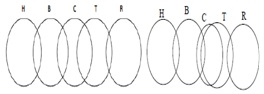So either I or III follows.
As I and III make a complementary pair.
DIRECTIONS for the question 5:  The question below is followed by two statements marked I and II. Mark as your answer.
1. if the data in statement I alone are sufficient to answer the question, while the data in statement II alone are not sufficient to answer the question.
2. if the data in statement II alone are sufficient to answer the question, while the data in statement I atone are not sufficient to answer the question.
3. if the data either in statement I alone or in statement II alone are sufficient to answer the question.
4. if the data given in both the statements I and II together are not sufficient to answer the question; and
5. if the data in both the statements 1 and II together are necessary to answer the question.
3. Statements: Among M, N, T, Q and D, who is the youngest?
I. T and D are younger than M.
II. Q is older than T but younger than D and N

Statement I lacks information regarding N and Q. Hence, I alone is not sufficient.
Statement II lacks information regarding M. Hence, II alone is not sufficient. Now, from I and II, we get that T is the youngest.
So data in both the statements I and II together are necessary to answer the question. Hence option E is the answer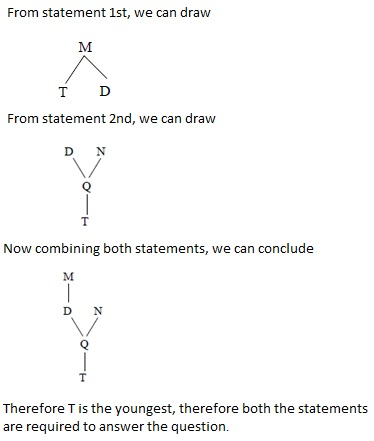DIRECTIONS for the question 6:  Solve the following question and mark the best possible option.
‘P # Q’ means ‘P is neither greater than nor equal to Q’.
‘P © Q’ means ‘P is neither equal to nor smaller than Q’.
‘P % Q’ means ‘P is neither smaller than nor greater than Q’.
‘P $Q’ means ‘P is not smaller than Q’. ‘P @ Q’ means ‘P is not greater than Q’. Now in each of the following questions, assuming the given statements to be true, find which of the three conclusions I, II and III given below them is/are definitely true and give your answer accordingly. 4. Statement: Z#F, R@F, D©R Conclusions: I. Z # R II.F#D III. D©Z 1. None is true 2. Only I is true 3. Only III is true 4. Only either I or III is true 5. All are true Answer: Option A Here we have Z < F …(i) R < F …(ii) D > R …(iii) From (i) and (ii), we can't relate Z with R; from (ii) and (iii) we can't relate F with D. Hence, neither conclusion I (Z < R) nor conclusion II (F < D) follows. On the basis of the given information, we can't get any specific conclusion regarding D and Z. Hence, conclusion III (D > Z) does not follow. Thus answer is 1st option. DIRECTIONS for the question 7 to 8:The question below has either two or three statements followed by two or three conclusions. You have to take the given statements to be true and then decide which of the given conclusions logically follows from the given statements, disregarding the commonly known facts. 5. Statements : All wasps are doors. No door is well. Conclusions : I. No wasp is well. II. No well is door. 1. If only conclusion I follows 2. If only conclusion II follows 3. If both conclusions follow 4. If neither of the two conclusions follows Answer: Option A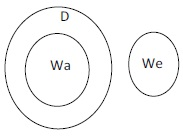So, both conclusion follow 6. Statements : All rocks are poles. No pole is a horse. Conclusions : I. Some rocks are horses. II. Some horses are pole 1. If only conclusion I follows 2. If only conclusion II follows 3. If both conclusions follow 4. If neither of the two conclusions follows Answer: Option D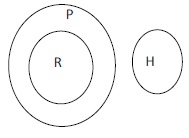So, no conclusions follow. DIRECTIONS for the question 9-10:Solve the following question and mark the best possible option. a) ‘x • y λ z’ means ‘z is multiplied by the sum of x and y’ b) ‘x$ y % z’ means ‘y is subtracted from x and the resultant is divided by z;.
c) ‘x # @ Y © z’ means ‘x is added to the resultant when y is divided by z’.
In each of the question below, a set of instruction sequences is given. You are required to find out the outcome which should come in place of the question mark (?) in each of the given sets of sequence
7. I. 120 \$ 40 % 8 = t II. t @ ? © 5 = 40
1. 100
2. 150
3. 200
4. 160
5. None of these
Answer: Option B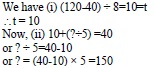Hence option B.
8. I. 48 # 12 * 8 = b II. b • 8 λ 5 =?
1. 200
2. 240
3. 260
4. 280
5. None of these